• 参考文章：最小二乘与交叉熵代价函数的区别（作用及公式推导）
参考文章：最小二乘与交叉熵代价函数的区别（作用及公式推导）
展开全文• 分别根据梯度法和最小二乘法求解多元函数问题分别使用梯度下降法和最小二乘法求解多元函数并进行比较，这里使用jupyter notebook平台进行Python编程一、题目描述二、使用梯度下降法求解多元函数（一）梯度下降法基本...


使用Python编程，分别根据梯度法和最小二乘法求解多元函数问题
分别使用梯度下降法和最小二乘法求解多元函数并进行比较，这里使用jupyter notebook平台进行Python编程一、题目描述二、使用梯度下降法求解多元函数（一）梯度下降法基本原理（二）梯度下降法公式（三）Python代码实现1、导入要使用到的库、定义变量并赋值2、代价函数3、使用梯度下降法求解多元函数系数4、打印系数和方程5、绘制线性回归方程拟合图6、完整代码7、运行结果

三、使用最小二乘法求解多元函数（一）最小二乘法基本原理（二）Python代码实现1、自行推导过程编写代码运行结果2、采用sklearn库运行结果

（四）将梯度下降法与最小二乘法进行对比

分别使用梯度下降法和最小二乘法求解多元函数并进行比较，这里使用jupyter notebook平台进行Python编程
一、题目描述
为求得某个地区的商品店的月营业额与店铺的面积、该店距离车站距离的相关性大小，以店铺面积、距离车站的距离、以及月营业额建立线性回归方程，并求解方程，得到相关系数。将表中的数据录入到Excel中，编程时会用到这些数据，如下：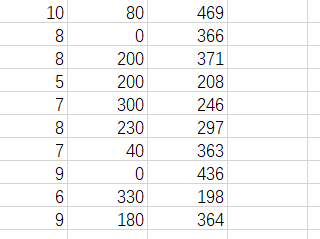二、使用梯度下降法求解多元函数
（一）梯度下降法基本原理
梯度下降法又称最速下降法，是求解无约束最优化问题的一种最常用的方法，在对损失函数最小化时经常使用。梯度下降法是一种迭代算法。选取适当的初值x(0)，不断迭代，更新x的值，进行目标函数的极小化，直到收敛。由于负梯度方向时使函数值下降最快的方向，在迭代的每一步，以负梯度方向更新x的值，从而达到减少函数值的目的。
（二）梯度下降法公式（三）Python代码实现
1、导入要使用到的库、定义变量并赋值
import numpy as np
from matplotlib import pyplot as plt
from mpl_toolkits.mplot3d import Axes3D
data=np.genfromtxt('D:/area_distance.csv',delimiter=',')
x_data=data[:,:-1]
y_data=data[:,2]
#定义学习率、斜率、截据
#设方程为y=theta1x1+theta2x2+theta0
lr=0.00001
theta0=0
theta1=0
theta2=0
#定义最大迭代次数，因为梯度下降法是在不断迭代更新k与b
epochs=10000

2、代价函数
def compute_error(theta0,theta1,theta2,x_data,y_data):
totalerror=0
for i in range(0,len(x_data)):#定义一共有多少样本点
totalerror=totalerror+(y_data[i]-(theta1*x_data[i,0]+theta2*x_data[i,1]+theta0))**2

3、使用梯度下降法求解多元函数系数
def gradient_descent_runner(x_data,y_data,theta0,theta1,theta2,lr,epochs):
m=len(x_data)
for i in range(epochs):
for j in range(0,m):
return theta0,theta1,theta2

4、打印系数和方程
theta0,theta1,theta2=gradient_descent_runner(x_data,y_data,theta0,theta1,theta2,lr,epochs)
print('迭代次数：{0} 学习率：{1}之后 a0={2},a1={3},a2={4},代价函数为{5}'.format(epochs,lr,theta0,theta1,theta2,compute_error(theta0,theta1,theta2,x_data,y_data)))
print("多元线性回归方程为:y=",theta1,"X1+",theta2,"X2+",theta0)

5、绘制线性回归方程拟合图
#画图
ax.scatter(x_data[:,0],x_data[:,1],y_data,c='r',marker='o')
x0=x_data[:,0]
x1=x_data[:,1]
#生成网格矩阵
x0,x1=np.meshgrid(x0,x1)
z=theta0+theta1*x0+theta2*x1
#画3d图
ax.plot_surface(x0,x1,z)
ax.set_xlabel('area')
ax.set_ylabel('distance')
ax.set_zlabel("Monthly turnover")
plt.show()

6、完整代码
import numpy as np
from matplotlib import pyplot as plt
from mpl_toolkits.mplot3d import Axes3D
data=np.genfromtxt('D:/area_distance.csv',delimiter=',')
x_data=data[:,:-1]
y_data=data[:,2]
#定义学习率、斜率、截据
#设方程为y=theta1x1+theta2x2+theta0
lr=0.00001
theta0=0
theta1=0
theta2=0
#定义最大迭代次数，因为梯度下降法是在不断迭代更新k与b
epochs=10000
#定义最小二乘法函数-损失函数（代价函数）
def compute_error(theta0,theta1,theta2,x_data,y_data):
totalerror=0
for i in range(0,len(x_data)):#定义一共有多少样本点
totalerror=totalerror+(y_data[i]-(theta1*x_data[i,0]+theta2*x_data[i,1]+theta0))**2
#梯度下降算法求解参数
m=len(x_data)
for i in range(epochs):
for j in range(0,m):
return theta0,theta1,theta2
#进行迭代求解
print('迭代次数：{0} 学习率：{1}之后 a0={2},a1={3},a2={4},代价函数为{5}'.format(epochs,lr,theta0,theta1,theta2,compute_error(theta0,theta1,theta2,x_data,y_data)))
print("多元线性回归方程为:y=",theta1,"X1+",theta2,"X2+",theta0)
#画图
ax.scatter(x_data[:,0],x_data[:,1],y_data,c='r',marker='o')
x0=x_data[:,0]
x1=x_data[:,1]
#生成网格矩阵
x0,x1=np.meshgrid(x0,x1)
z=theta0+theta1*x0+theta2*x1
#画3d图
ax.plot_surface(x0,x1,z)
ax.set_xlabel('area')
ax.set_ylabel('distance')
ax.set_zlabel("Monthly turnover")
plt.show()

7、运行结果三、使用最小二乘法求解多元函数
（一）最小二乘法基本原理
在我们研究两个变量（x,y）之间的相互关系时，通常可以得到一系列成对的数据（x1,y1.x2,y2… xm,ym）；将这些数据描绘在x -y直角坐标系中，若发现这些点在一条直线附近，可以令这条直线方程为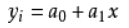（式1-1） 其中：a0、a1 是任意实数 为建立这直线方程就要确定a0和a1，应用《最小二乘法原理》，将实测值Yi与利用计算值Yj（Yj=a0+a1Xi）（式1-1）的离差（Yi-Yj）的平方和 最小为“优化判据”。 令：φ = （式1-2) 把（式1-1）代入（式1-2）中得： φ = （式1-3) 当 最小时，可用函数 φ 对a0、a1求偏导数，令这两个偏导数等于零。 ∑2(a0 + a1Xi - Yi）=0（式1-4) ∑2Xi（a0 +a1Xi - Yi）=0（式1-5) 亦即： na0 + （∑Xi ) a1 = ∑Yi （式1-6) （∑Xi ) a0 + （∑Xi^2 ) a1 = ∑（Xi*Yi) （式1-7) 得到的两个关于a0、 a1为未知数的两个方程组，解这两个方程组得出： a0 = （∑Yi) / n - a1（∑Xi) / n （式1-8) a1 = [n∑(Xi Yi) - （∑Xi ∑Yi)] / (n∑Xi^2 -∑Xi∑Xi)（式1-9) 这时把a0、a1代入（式1-1）中， 此时的(式1-1）就是我们回归的一元线性方程即：数学模型。 在回归过程中，回归的关联式不可能全部通过每个回归数据点（x1,y1. x2,y2…xm,ym），为了判断关联式的好坏，可借助相关系数“R”，统计量“F”，剩余标准偏差“S”进行判断；“R”越趋近于 1 越好；“F”的绝对值越大越好；“S”越趋近于 0 越好。 R = [∑XiYi - m （∑Xi / m）（∑Yi / m)]/ SQR{[∑Xi2 - m （∑Xi / m)2][∑Yi2 - m （∑Yi / m)2]} （式1-10) * 在（式1-10）中，m为样本容量，即实验次数；Xi、Yi分别为任意一组实验数据X、Y的数值。
（二）Python代码实现
1、自行推导过程编写代码
import numpy as np
import matplotlib.pyplot as plt
import pandas as pd
import seaborn as sns

%matplotlib inline
data = np.genfromtxt("D:/area_distance.csv",delimiter=",")
X1=data[0:10,0]#面积
X2=data[0:10,1]#离车站的距离
Y=data[0:10,2]#月营业额
#将因变量赋值给矩阵Y1
Y1=np.array([Y]).T
#为自变量系数矩阵X赋值
X11=np.array([X1]).T
X22=np.array([X2]).T
A=np.array([,,,,,,,,,])#创建系数矩阵
B=np.hstack((A,X11))#将矩阵a与矩阵X11合并为矩阵b
X=np.hstack((B,X22))#将矩阵b与矩阵X22合并为矩阵X
#求矩阵X的转置矩阵
X_=X.T
#求矩阵X与他的转置矩阵的X_的乘积
X_X=np.dot(X_,X)
#求矩阵X与他的转置矩阵的X_的乘积的逆矩阵
X_X_=np.linalg.inv(X_X)
#求解系数矩阵W，分别对应截距b、a1、和a2
W=np.dot(np.dot((X_X_),(X_)),Y1)
b=W
a1=W
a2=W
print("系数a1={:.1f}".format(a1))
print("系数a2={:.1f}".format(a2))
print("截距为={:.1f}".format(b))
print("多元线性回归方程为:y={:.1f}".format(a1),"X1+ {:.1f}".format(a2),"X2+{:.1f}".format(b))
#画出线性回归分析图
sns.pairplot(data1, x_vars=['area','distance'], y_vars='Y', height=3, aspect=0.8, kind='reg')
plt.show()
#求月销售量Y的和以及平均值y1
sumy=0#因变量的和
y1=0#因变量的平均值
for i in range(0,len(Y)):
sumy=sumy+Y[i]
y1=sumy/len(Y)
#求月销售额y-他的平均值的和
y_y1=0#y-y1的值的和
for i in range(0,len(Y)):
y_y1=y_y1+(Y[i]-y1)
print("营业额-营业额平均值的和为:{:.1f}".format(y_y1))
#求预测值sales1
sales1=[]
for i in range(0,len(Y)):
sales1.append(a1*X1[i]+a2*X2[i]+b)
#求预测值的平均值y2
y2=0
sumy2=0
for i in range(len(sales1)):
sumy2=sumy2+sales1[i]
y2=sumy2/len(sales1)
#求预测值-平均值的和y11_y2
y11_y2=0
for i in range(0,len(sales1)):
y11_y2=y11_y2+(sales1[i]-y2)
print("预测营业额-预测营业额平均值的和为:{:.1f}".format(y11_y2))
#求月销售额y-他的平均值的平方和
Syy=0#y-y1的值的平方和
for i in range(0,len(Y)):
Syy=Syy+((Y[i]-y1)*(Y[i]-y1))
print("Syy={:.1f}".format(Syy))
#求y1-y1平均的平方和
Sy1y1=0
for i in range(0,len(sales1)):
Sy1y1=Sy1y1+((sales1[i]-y2)*(sales1[i]-y2))
print("Sy1y1={:.1f}".format(Sy1y1))
#（y1-y1平均）*（y-y平均）
Syy1=0
for i in range(0,len(sales1)):
Syy1=Syy1+((Y[i]-y1)*(sales1[i]-y2))
print("Syy1={:.1f}".format(Syy1))
#求y-y1的平方Se
Se=0
for i in range(0,len(sales1)):
Se=Se+((Y[i]-y2)*(Y[i]-y2))
print("Se={:.1f}".format(Se))
#求R
R=Syy1/((Syy*Sy1y1)**0.5)
R2=R*R
print("R2={:.4f}".format(R2))

运行结果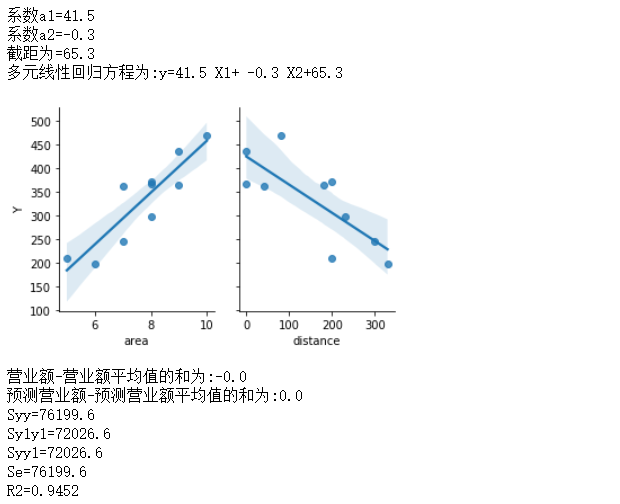2、采用sklearn库
import pandas as pd
import seaborn as sns
import matplotlib.pyplot as plt
from sklearn import linear_model
%matplotlib inline

X=data[['area','distance']]           #x,y取值
y=data['Y']
y=data.Y
model = linear_model.LinearRegression()
model.fit(X,y)
print("系数a1={:.1f}".format(model.coef_))
print("系数a2={:.1f}".format(model.coef_))
print("截距为={:.1f}".format(model.intercept_))
print("多元线性回归方程为:y={:.1f}".format(model.coef_),"X1+ {:.1f}".format(model.coef_),"X2+{:.1f}".format(model.intercept_))
sns.pairplot(data, x_vars=['area','distance'], y_vars='Y', height=3, aspect=0.8, kind='reg')
plt.show()

运行结果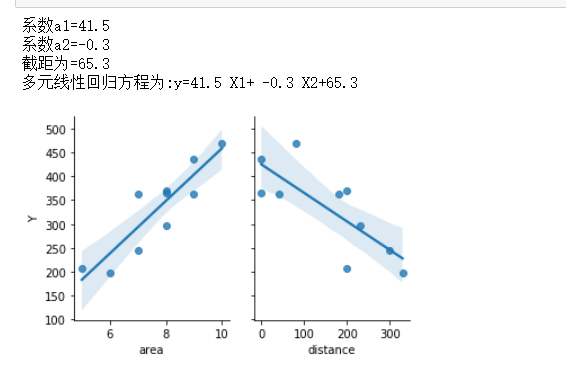（四）将梯度下降法与最小二乘法进行对比
这里给出利用Excel表格的数据分析功能得出的数据：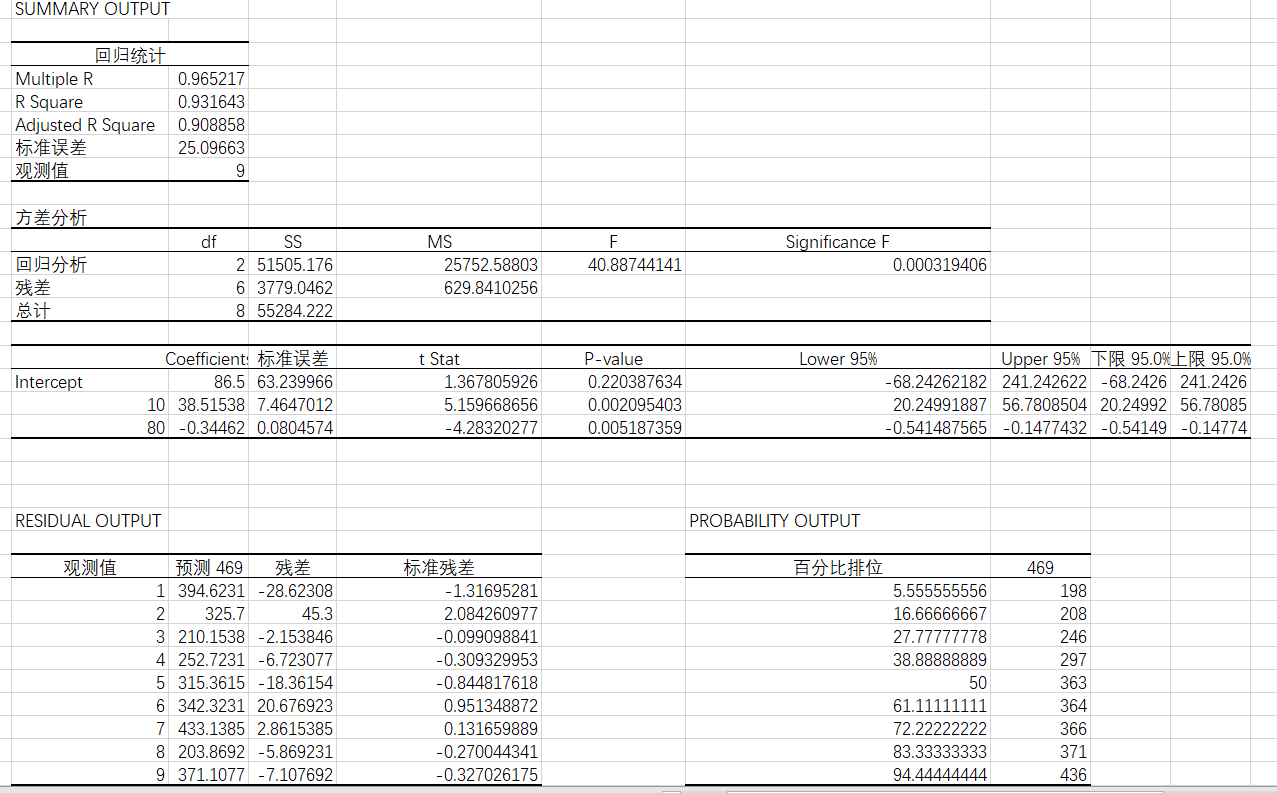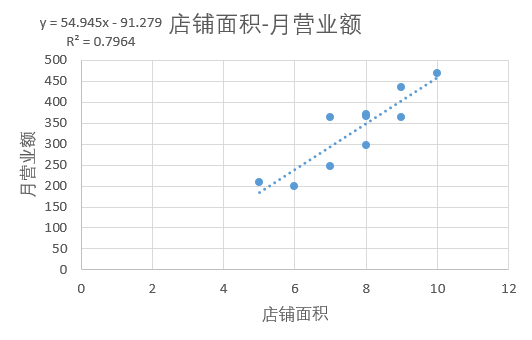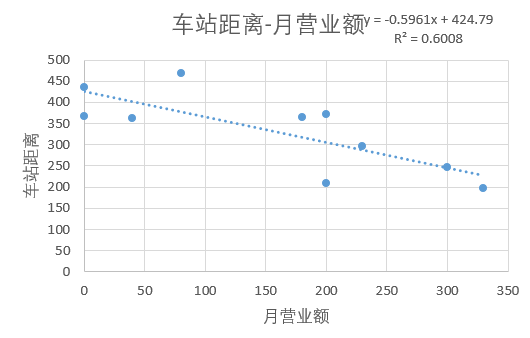通过对两种方法的对比分析，不难得出结论：最小二乘法计算量偏大，而且求逆矩阵相当耗费时间，求逆矩阵也会存在数值不稳定的情况，相比之下梯度下降法可以看做是一种更简单的最小二乘法最后一步解方程的方法，梯度下降法虽然有一定的缺点，但是计算量不大，在数据量比较大的时候选择梯度下降法比较好一点。
展开全文机器学习
• 梯度下降目的是最小化损失函数： 批量梯度下降（Batch Gradient Descent，BGD） 优点： （1）准确率高。 缺点： （1）训练和收敛速度慢。 （2）可能不会收敛到最小值，而是在附近震荡。解决方法：采用学习率衰减的...
前言
梯度下降目的是最小化损失函数：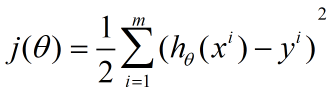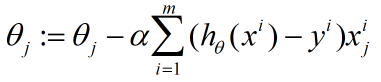优点： （1）准确率高。 缺点： （1）训练和收敛速度慢。 （2）可能不会收敛到最小值，而是在附近震荡。解决方法：采用学习率衰减的方法。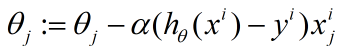优点：   （1）在每轮迭代中，随机优化某一条训练数据上的损失函数，训练速度快。 缺点：   （1）准确度不行。   （2）可能会收敛到局部最优。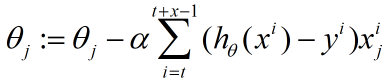优点：   （1）一个batch上优化神经网络参数并不会比单个数据慢太多。   （2）每次使用一个batch可以大大减小收敛所需要的迭代次数，同时可以使收敛到的结果更加接近梯度下降的效果。 缺点：   （1）batch_size的选择需要多次选择才能得到比较优秀的结果。
代价函数的解释
假设输入与 目标变量关系：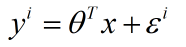右侧第二项是线性模型未捕捉到的因素，假设为独立同分布符合正态分布，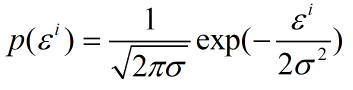那么有：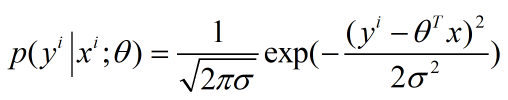引入似然函数：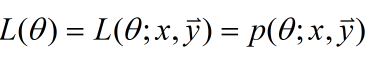因为是独立发布的，那么所有的样本的概率就是每个样本的概率和：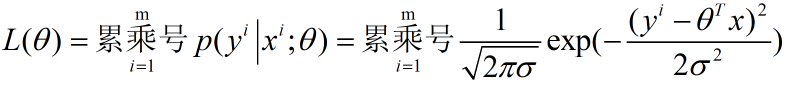(实在没有在mathtype里找到累乘号…) 再引入似然对数：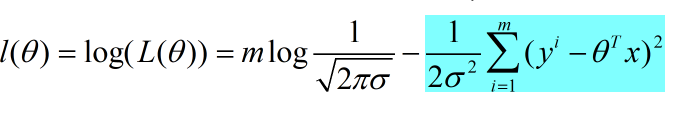带蓝标的就是我们的得到的优化目标。
与其他无约束优化算法比较
（1）最小二乘法 a:梯度下降需要选择步长而他不要 b:梯度下降是迭代求解，最小二乘法是求得解析解 c:样本多就梯度下降，较小时最小二乘法解 定义损失函数：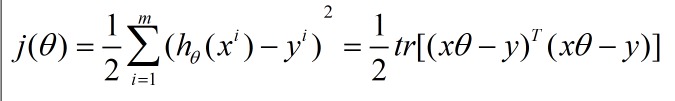直接求导，求最小值：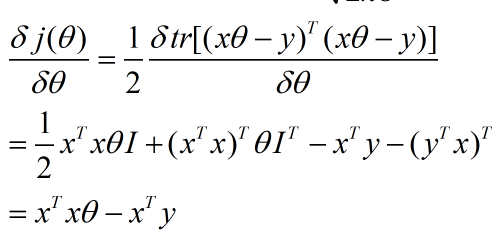当样本M很少，小于特征数N的时候，这时拟合方程是欠定的需要使用LASSO。】 当M=N，可以用方程组求解。 当M大于N时候，拟合方程过定，可以使用最小二乘法。
局限性： 1）：需要计算逆矩阵，但有时候逆矩阵不一定存在。 2）：当特征数特别大，计算耗时，建议不超过10000个特征。 3）：如果拟合函数非线性，无法使用。
（2）牛顿法 a:都是迭代求解 b:梯度下降是梯度求解，而牛顿法用二阶海森伪矩阵求解 c:牛顿法收敛更快，但迭代时间 题梯度下降要长。
后续会更新：牛顿法，阻尼牛顿法，拟牛顿法，DFP, BFGS等相关介绍。 已更新：https://blog.csdn.net/weixin_45232469/article/details/98035576
展开全文• 最小二乘法是一种相对来说比较简单而且易于理解的算法，在分类回归算法中经常使用。最近在学习Deep Learning这本书，遇到线性最小二乘，刚开始对于书本上的公式还不是很理解，后来经过查阅资料，对线性最小二乘的...
        最小二乘法是一种相对来说比较简单而且易于理解的算法，在分类回归算法中经常使用。最近在学习Deep Learning这本书，遇到线性最小二乘，刚开始对于书本上的公式还不是很理解，后来经过查阅资料，对线性最小二乘的原理以及如何使用有了一定的理解，话不多说，直接步入正题。
一、最小二乘法

官方定义：最小二乘法（又称最小平方法）是一种数学优化技术。它通过最小化误差的平方和寻找数据的最佳函数匹配。利用最小二乘法可以简便地求得未知的数据，并使得这些求得的数据与实际数据之间误差的平方和为最小。最小二乘法还可用于曲线拟合。其他一些优化问题也可通过最小化能量或最大化熵用最小二乘法来表达。
我的理解：最小二乘法就是最小化代价函数（损失函数），如果最后所求得的目标函数在图像上显示过拟合，则可以通过添加正则化项来进行降拟合

下面通过一个直观的栗子来介绍一下最小二乘法是怎么发挥作用的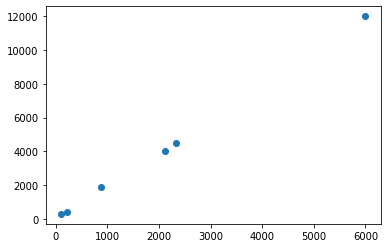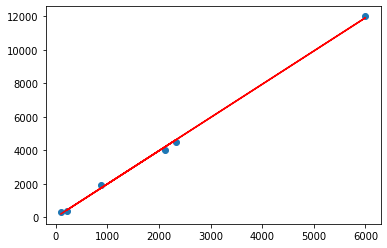由图1-->图2就是通过最小二乘法拟合出一条直线，这条直线表示所要求得的数据与真实数据之间误差平方和最小的目标函数
二、最小二乘法的原理
假设我们现在有一系列的数据点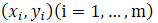,由我们所给出的拟合函数h(x),所得到的估量就是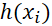，那么如何评估我们所给出的拟合函数与实际待求解的函数的拟合程度呢？这里先引入一个概念：残差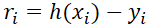，评估拟合程度就是在残差的基础之上进行的。
由以上的认知，我们就可以利用公式来表示最小二乘法了：对于给定的数据，在取定的假设空间中求解h(x)∈H，使得残差的2-范式最小，也就是使得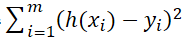的值最小。从几何上讲，就是寻找与给定点距离平方和最小的函数y = h(x)，在上面的两张图中很容易理解这句话。
那么最小二乘法如何求解呢？假设我们拟合一个线性函数h(x, w) = w0 + w1 * x（当然也可以是二次函数或者是更高维的函数），假设我们的目标就是要找这样的函数w，使得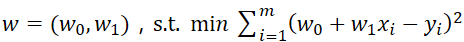，这里的意思是寻找一个目标函数w，使得损失函数最小。这里令损失函数为Q(w)，即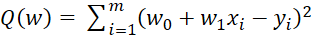，可以用微积分的知识来求解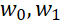，这是一个典型的求极值的问题，分别对求偏导数，然后令偏导数等于零，即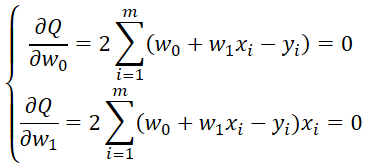求解这个方程便可求解出三、最小二乘法实例
这里我们把目标函数选为y=sin(2πx)
import numpy as np  # 引入numpy
import scipy as sp
import pylab as pl
from scipy.optimize import leastsq  # 引入最小二乘函数

n = 9  # 多项式次数
# 目标函数
def real_func(x):
return np.sin(2 * np.pi * x)

# 多项式函数
def fit_func(p, x):
f = np.poly1d(p)
return f(x)

# 残差函数
def residuals_func(p, y, x):
ret = fit_func(p, x) - y
return ret

x = np.linspace(0, 1, 9)  # 随机选择9个点作为x
x_points = np.linspace(0, 1, 1000)  # 画图时需要的连续点

y0 = real_func(x)  # 目标函数
y1 = [np.random.normal(0, 0.1) + y for y in y0]  # 添加正太分布噪声后的函数
p_init = np.random.randn(n)  # 随机初始化多项式参数
plsq = leastsq(residuals_func, p_init, args=(y1, x))
print (plsq)  # 输出拟合参数
pl.plot(x_points, real_func(x_points), label='real')
pl.plot(x_points, fit_func(plsq, x_points), label='fitted curve')
pl.plot(x, y1, 'bo', label='with noise')
pl.legend()
pl.show()
输出结果为：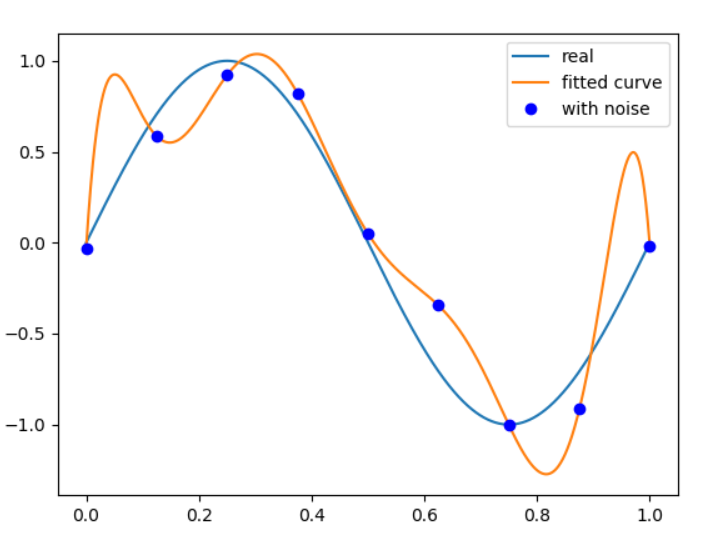由图可以看出我们拟合的函数过拟合了，下面我们尝试在损失函数的基础上加上正则化项，来降低过拟合的现象：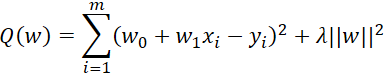# 残差函数
regularization = 0.0001  # 正则化系数lambda

# 残差函数
def residuals_func(p, y, x):
ret = fit_func(p, x) - y
ret = np.append(ret, np.sqrt(regularization) * p)   # 将lambda^(1/2)p加在了返回的array的后面
return ret
输出的图像为：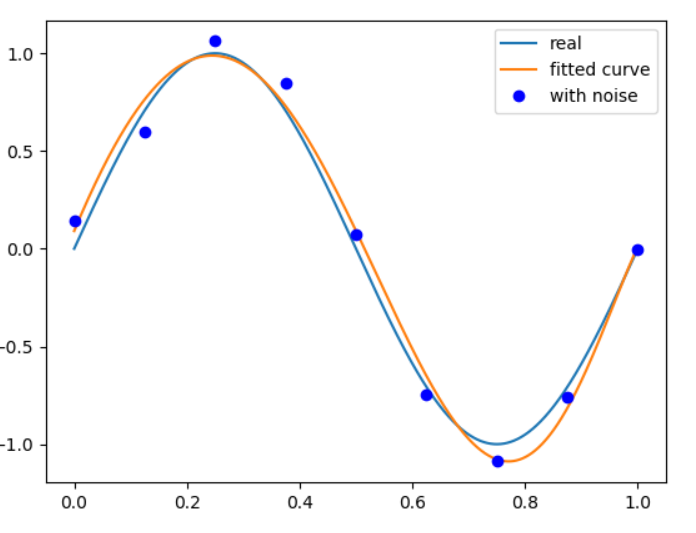这里要注意如果正则化项的系数太大，会导致欠拟合现象（此时的惩罚项权重特别高）

展开全文深度学习
• 前言废话     前面讲了线性回归分析，从观测数据中确定线性...1、多元函数求最值（也有的说法把这当成最小二乘法） 2、利用线代的几何意义 3、梯度下降法 一、多元函数求最值 从本质上来说，上述均方误差和就是...数学模型 线性回归
• 岭回归： 以损失部分信息(说明加入正则化项确实有深刻的缺点)、降低精度(加入正则化项)为代价获得回归系数更为符合实际、更可靠的回归方法，对病态数据的拟合要强于最小二乘法。实际上也是新瓶装旧酒，都一样的。 ...1024程序员节
• 最小二乘法 一、什么是最小二乘法 最小二乘法作为一种数学优化技术，通过最小化误差的平方和寻找数据的最佳函数匹配。（重点：寻找匹配函数） ...二、代价函数Cost function 根据最小二乘法的...
• 即最小化代价函数: 　（其中：m为训练样本的数量） 　（1）最小二乘法： 　（2）梯度下降法： 　开始时我们随机选择一个参数的组合（θ0,θ1,...,θn），计算代价 函数，然后我们寻找下一个能让代价函数值...
• 最小二乘法 最小二乘法是我们经常用到的求解模型的回归方法，是一种优化方法，对于一个线性模型 Xθ=yXθ=yX\theta=y XXX为mmm行nnn列的矩阵，代表mmm个样本，每个样本有nnn个变量，即有mmm个等式，未知数nnn个，...主成分分析 矩阵的迹 无偏估计
• ## 普通最小二乘法

千次阅读 2018-08-02 23:59:57
sklearn中的LinearRegression类拟合了一个带有系数w=(w1,w2,...,wp)w=(w1,w2,...,wp)w=(w_1,w_2,...,w_p)的线形模型，使得数据集实际观测数据和预测数据之间的残差平方和最小： minw||Xw−y||22minw||Xw−y||22min_...机器学习 线性回归
• 最小二乘法的核心思想直接计算出使得代价函数的梯度（偏导数）为零的参数向量。其优点就是不用一步步的调整参数，而是直接求解出的最优的参数。线性回归 多项式回归 机器学习
• 使用梯度下降法求多元函数的系数并与最小二乘法进行比较梯度下降法求解多元函数的系数最小二乘法求解多元函数的系数两者的比较和总结 梯度下降法求解多元函数的系数 最小二乘法求解多元函数的系数 两者的比较和总结 ...python 机器学习
• 机器学习-线性回归线性回归背景最小二乘法计算线性回归数据的准备把数据转换成数学损失函数根据损失函数计算最终结果总结 最近老师让我学习线性回归编程模型，此篇介绍使用最小二乘法实现单机器线性回归。 线性回归...线性回归 公式推导 机器学习
• ## 最小二乘法

千次阅读 2015-08-16 22:31:11
的时候，普通最小二乘法和数据最小二乘需要满足两个基本假设 ：1 数据矩阵 A A 非奇异或者满列秩；2 数据向量 b b 或者数据矩阵 A A 存在加性噪声或误差。 Tikhonov 正则化最小二乘方法就是为了解决数据...矩阵论 数据 统计学
• 线性回归-最小二乘法概述给定包含正确答案的样本数据集，选择一个概念函数H，并为H找到适应样本数据集的最优参数，从而建立预测模型为新查询数据预测答案。线性最小二乘法原理对于已知的样本数据集，可以用线性方程...机器学习 线性回归
• 先选取最为简单的一元线性函数拟合助于我们理解最小二乘法的原理。 要让一条直接最好的拟合红色的数据点，那么我们希望每个点到直线的残差都最小。 设拟合直线为 yi=b+axiy_{i}= b+ax_{i}yi​=b+axi​,那这些数据的...机器学习 梯度下降机器学习 逻辑回归 python
• 讲到这里又需要引入一个新的概念：损失函数（代价函数） 在机器学习中，我们希望找到一个面向整个模型的度量函数，使得这个度量函数越小越好。 本来我们求的是似然函数的最大值，现在把问题转换成了求目标函数的...
• 并为变量赋值2、定义系数初始值以及学习率和迭代次数3、定义最小二乘法函数-损失函数（代价函数）4、定义梯度下降算法求解线性回归方程系数python函数5、代用函数，进行系数求解，并打印6、画出回归方
• 在线性回归算法求解中，常用的是最小二乘法与梯度下降法，其中梯度下降法是最小二乘法求解方法的优化，但这并不说明梯度下降法好于最小二乘法，实际应用过程中，二者各有特点，需结合实际案例具体分析。 最后有两份...
• 1 LDA与最小二乘法的关系 2.LDA的变种详解LDA
• 回归问题的前提：1） 收集的数据2） 假设的模型，即一个函数，这个函数里含有未知的参数，通过学习，可以估计出参数。然后利用这个模型去预测/分类新的数据。1. 线性回归假设 特征 和 结果 都满足线性。即不大于一次...
• 梯度下降法 ...批量梯度下降法：将所有样本代价函数的和除以样本数量作为代价函数。然后求偏导数进行迭代计算。 附上代码，不知道实现的对不对。 #include int main() { cout ; return 0 ; }
• 1.普通最小二乘法（Ordinary Least Squares） 线性回归的目的就是是的预测值与实际值的残差平方和最小： import matplotlib.pyplot as plt import numpy as np #载入数据集“datasets” from sklearn import datapython 回归
• * @return 返回一个CostFunction*，一直没想出CostFunction的很贴切的名字，代价函数太直白不够形象，这里其实有点导函数的意思，但名字上又完全不相关 */ static ceres :: CostFunction * Create ( const ......